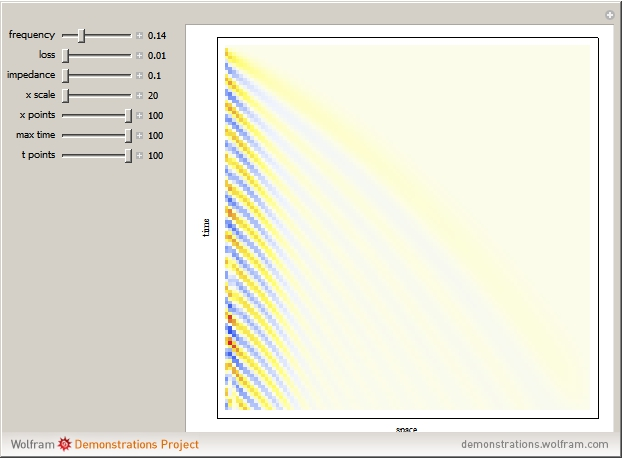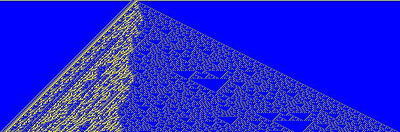#Wolfram Summer School

# Alumni# Soumyajit Mandal

Summer School

Class of 2008

## Bio

Soumyajit Mandal is a graduate student at MIT in electrical engineering and computer science. One of his main interests is novel ways of performing computation, particularly in biological systems and using distributed active media. Currently he is working on circuits that emulate the spectral decomposition of sound in the cochlea (inner ear), but at radio frequencies instead of audio. He is also doing some work on hardware models of biochemical networks. In the past he has worked on communication systems using chaos, analog, mixed-signal and RF circuit design, biomedical systems, and antennas.

## Project: Exploring Nonlinear Partial Differential Equations

This project is to find partial differential equations (PDEs) that can be implemented in some physical medium and used for fast distributed computation. An example is the spatially varying wave equation that is used by the mammalian cochlea to decompose input sounds into their constituent frequency components. Nonlinear PDEs of the first and second order will be investigated, beginning with those that have two independent variables (one space and one time dimension). A good example is the wave equation (an example of bizarre behavior exhibited by a nonlinear wave equation may be found on pages 165 and 166 of the NKS book). Hyperbolic equations like the wave equation have the nice property that information only travels at a given finite wave speed, meaning that specifying initial conditions is sufficient for finding solutions at later times. Other boundary conditions can be largely ignored. Another class of systems that will be looked at is reaction-diffusion equations with three or more variables, which appear to have received relatively little attention but are known to be capable of generating a wide range of complex spatio-temporal patterns.

The main reason nonlinearities are important is that they are unavoidable when there are any active processes in the system. Active processes, which require energy inputs to function, are necessary in order for any PDE to be used for computational purposes: a simple passive system is basically dead and decays to equilibrium in an uninteresting way. The goal is to discover interesting PDE behavior by adding simple generic nonlinearities of various types to hitherto linear equations, group these behaviors into classes, and then analyze them.

## Project-Related Demonstrations

### A Passive Cochlear Model